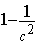### Seeing is believing!

Before you order, simply sign up for a free user account and in seconds you'll be experiencing the best in CFA exam preparation.

##### Subject 7. Chebyshev's Inequality
The Russian mathematician, Pafnuty Chebyshev, developed a useful theorem of inequality dealing with standard deviation as a measure of dispersion.

Let c be any number greater than 1. For any sample or population of data, the proportion of observations that lie FEWER than c standard deviations from the mean is at least:• This applies to both populations and samples, and to discrete and continuous data, regardless of the shape of the distribution.
• It gives a conservative estimate of the proportion of observations in an interval around the mean.
• It highlights the importance of σ and places lower and upper limits.
• Empirical rule: 68% within 1σ, 95% within 2σ, and 99% within 3σ.

Chebyshev's Inequality theorem is useful in that if we know the standard deviation, we can use it to measure the minimum amount of dispersion.

Example

What approximate percent of a distribution will lie within ± two standard deviations of the mean?
Chebyshev's Inequality: 1-(1/c2) = 1 - (1/22) = 0.75 or 75%

Several other values are also useful to know.

• A minimum of 36% of observations lie within 1.25 σ of the mean.
• A minimum of 56% of observations lie within 1.5 σ of the mean.
• A minimum of 75% of observations lie within 2 σ of the mean.
• A minimum of 84% of observations lie within 2.5 σ of the mean.
• A minimum of 89% of observations lie within 3 σ of the mean.
• A minimum of 94% of observations lie within 4 σ of the mean.

Learning Outcome Statements

h. calculate and interpret the proportion of observations falling within a specified number of standard deviations of the mean using Chebyshev's inequality;

CFA® 2021 Level I Curriculum, , Volume 1, Reading 7

User Comment
sarath Important concept.
itconcepts why does the empirical rule differ from the "other useful values to know"
achu empirical rule shows the dispersion of a normal distribution (within 1 sd =68%, 2sds 95%). As you can see, the normal distribution dispersion is 'tighter' than that shown by Cheby's inequality, but this is fine. At least 75% must be within 2sd's, but in a normal dist it goes up to 95%.
timmak I would say any entry level university textbook on stats and probability should cover this topic in more detail.
Nuta You can find good practical examples for understanding if you refer to 6 sigma rule.
Sophorior Empirical rule: 68% within 1 sd;, 95% within 2 sd;, and 99% within 3 sd. this is valid for a normal distribution, not for any distribution (so it shoudn't be mentioned here...)
tschorsch is this not a limit of maximum dispersion?
i.e. regardless of the distribution, no more than 1/k² of the values can be more than k std deviations from the mean
Ryan1234 That is correct.
mydogo I found this to a much simpler explanation to remember and apply, thus good for retention.